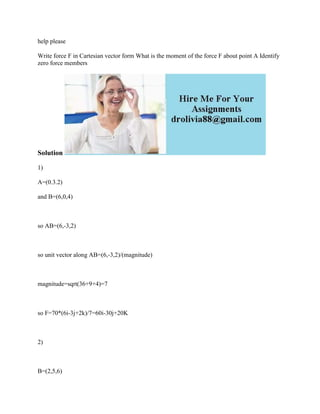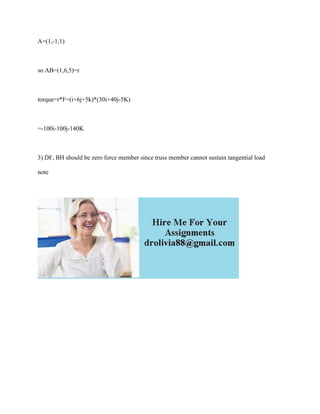Diese Präsentation wurde erfolgreich gemeldet.

# help please Write force F in Cartesian vector form What is the moment.docx

Anzeige
Anzeige
Anzeige
Anzeige
Anzeige
Anzeige
Anzeige
Anzeige
Anzeige
Anzeige
Anzeige×

1 von 2 Anzeige

# help please Write force F in Cartesian vector form What is the moment.docx

Write force F in Cartesian vector form What is the moment of the force F about point A Identify zero force members
Solution
1)
A=(0.3.2)
and B=(6,0,4)

so AB=(6,-3,2)

so unit vector along AB=(6,-3,2)/(magnitude)

magnitude=sqrt(36+9+4)=7

so F=70*(6i-3j+2k)/7=60i-30j+20K

2)

B=(2,5,6)
A=(1,-1,1)

so AB=(1,6,5)=r

torque=r*F=(i+6j+5k)*(30i+40j-5K)

=-100i-100j-140K

3) DF, BH should be zero force member since truss member cannot sustain tangential load
note

.

Write force F in Cartesian vector form What is the moment of the force F about point A Identify zero force members
Solution
1)
A=(0.3.2)
and B=(6,0,4)

so AB=(6,-3,2)

so unit vector along AB=(6,-3,2)/(magnitude)

magnitude=sqrt(36+9+4)=7

so F=70*(6i-3j+2k)/7=60i-30j+20K

2)

B=(2,5,6)
A=(1,-1,1)

so AB=(1,6,5)=r

torque=r*F=(i+6j+5k)*(30i+40j-5K)

=-100i-100j-140K

3) DF, BH should be zero force member since truss member cannot sustain tangential load
note

.

Anzeige
Anzeige

### help please Write force F in Cartesian vector form What is the moment.docx

1. 1. help please Write force F in Cartesian vector form What is the moment of the force F about point A Identify zero force members Solution 1) A=(0.3.2) and B=(6,0,4) so AB=(6,-3,2) so unit vector along AB=(6,-3,2)/(magnitude) magnitude=sqrt(36+9+4)=7 so F=70*(6i-3j+2k)/7=60i-30j+20K 2) B=(2,5,6)
2. 2. A=(1,-1,1) so AB=(1,6,5)=r torque=r*F=(i+6j+5k)*(30i+40j-5K) =-100i-100j-140K 3) DF, BH should be zero force member since truss member cannot sustain tangential load note